# JavaScript : Logical Operators – Theory

Objectives

1. Learn to recognize a Boolean expression;
2. Understand the 3 logical operators;
3. Be able to construct a Boolean expression using the logical operators appropriately.

Definition

A Boolean expression is a sequence of items that ultimately lead to a result that is true or false.

Listed below are the main comparison operators and several Boolean expressions with their results:

== Equal to Not equal to Greater than Less than Greater than or equal to Less than or equal to
• - 2 == 3 always returns the result false;
• - 2 != 3 always returns the result true;
• - 2 + 2 == 4 always returns the result true;
• - It's raining returns a result that may be true or false depending on meteorological conditions.

You have already used Boolean expressions in previous exercises without realizing it:

• - tails() checks whether the visible side of the coin is tails (true) or not (false);
• - detourLeft() checks whether there is a detour to the left or not;
• - diamondsCard() checks whether the card drawn is diamonds or not;
• - blueBall() checks whether the ball caught is blue or not.

At this point, you have already mastered the basic Boolean expressions. Of course, it's no fun if it’s too easy :O) ... So we will take things a step further, and look at how to manipulate and combine basic Boolean functions. This is done using the 3 logical operators presented below.

The 3 Logical Operators

1. The Logical Operator AND (&&)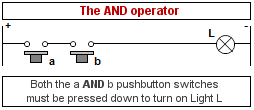As shown in this drawing, in order to make the light come on (i.e., for the result of the expression to be true), both the a and b buttons must be pressed simultaneously (pressing on a must be true AND pressing on b must be true). In all other cases, the lamp will remain off (the result of the expression will be false).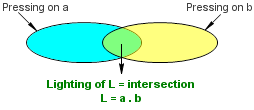We can see that when Lamp L lights up, it occurs as the intersection of the times when both a and b are pressed down.

In JavaScript, the syntax looks like this:

if ( test_1() && test_2() ) ...

2. The Logical Operator OR (||)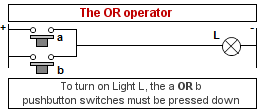As shown in this drawing, for the light to come on (for the result of the expression to be true), either pushbuttons a or b must be pushed (pressing on a must be true OR pressing on b must be true). The lamp will also come on if both pushbuttons are pressed down at the same time. If neither pushbutton is pressed down, the lamp will remain off (the result of the expression will be false).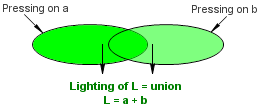Note that the sum of the times Lamp L lights up is the union of the times a is pressed down and the times b is pressed down.

In JavaScript, the syntax looks like this:

if ( test_1() || test_2() ) ...

3. The Logical Operator NOT (!)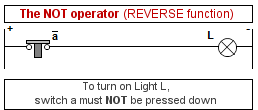As shown in this drawing, in order for the light to come on (for the result of the expression to be true), Pushbutton a must NOT be pressed down (i.e., pressing on a must be false). This operator is called the reverse or negative operator since the state of the lamp is the opposite of the state of the pushbutton.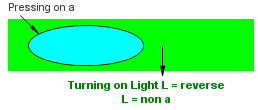Note that the sum of the times Lamp L lights up is the opposite of the times a is pressed down.

In JavaScript, the syntax looks like this:

if ( !test_1() ) ...

Constructing Boolean Expressions

The objective of the next 5 exercises is to give you situations in which to construct Boolean expressions and make use of these logical operators.

Good luck!

Back